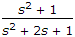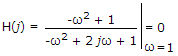# Electronics and Communication Engineering - Exam Questions Papers - Discussion

Discussion Forum : Exam Questions Papers - Exam Paper 10 (Q.No. 40)
40.
An LTI system having transfer functionand input x(t) = sin (t + 1) is in steady state. The output is sampled at a rate ωs rad/s to obtain the final output {y(k)}. Which of the following is true?
y(.) is zero for all sampling frequencies ωs
y(.) is nonzero for all sampling frequencies ωs
y(.) is nonzero for ωs > 2, but zero for ωs > 2
y(.) is zero for ωs > 2 but nonzero for ωs > 2
ω = 1,Thus output is zero for all sampling frequencies.